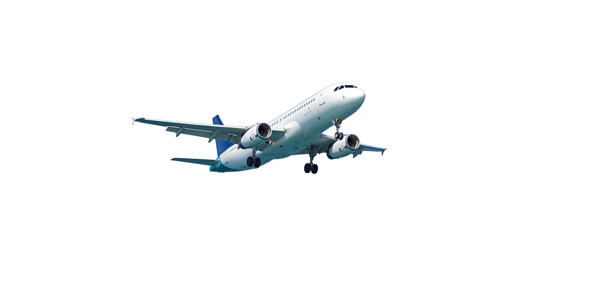# Tecnam P92-js Aircraft Flight Manual Exam

30 Questions | Total Attempts: 106SettingsFor those of you who would like to know more about and all things aircrafts plus things that led to various events in the aircraft filed, this is for you. If you are prepared to test your knowledge try it out.

Related Topics
• 1.
Aircraft has a:
• A.

Full JAA type certification

• B.

Is type certified under JAA as a VLA (very light aircraft)

• C.

Is type certified under JAA as a LSA (light sports aircraft)

• D.

Is type certified by the Latvian CAA

• 2.
Aircraft has:
• A.

1.5 degree dihedral

• B.

1.5 degree anhedral

• C.

No dihedral / anhedeal

• D.

91.5 degree dihedral

• 3.
Engine is manufacrured by:
• A.

Lycoming

• B.

Continental

• C.

Rotax GmbH

• D.

Bombardier-Rotax GmbH

• 4.
Engine model is:
• A.

912 S2

• B.

912

• C.

912 UL

• D.

IO-240B

• 5.
Cylinders in the engine are arranged in:
• A.

• B.

V-shape

• C.

In-line

• D.

Horizontally opposed

• 6.
Engine has:
• A.

2 cylinders

• B.

4 cylinders

• C.

6 cylinders

• D.

8 cylinders

• 7.
Engine is:
• A.

Liquid cooled

• B.

Air cooled

• C.

Cooled by air and cooling is aided by the use of cowl flaps

• D.

Liquid and air cooled

• 8.
Maximum power (less than 5 minutes) of the engine is:
• A.

98.6 hp

• B.

121 hp

• C.

88.5 hp

• D.

100 hp

• 9.
Maximum continuous power is:
• A.

92.5hp

• B.

51.5hp

• C.

100hp

• D.

105.5hp

• 10.
Engine can be run at maximum continuous power for:
• A.

A maximum of 5 minutes

• B.

A maximum of 7 minutes

• C.

As long as oil temperature, cylinder head temperature and oil pressure remain bellow specific values

• D.

A maximum of 3 minute

• 11.
Propeller is:
• A.

• B.

• C.

• D.

A variable pitch propeller

• 12.
Standard empty weight of the aircraft is:
• A.

550 kg

• B.

325 kg

• C.

225 kg

• D.

358 kg

• 13.
You may refuel
• A.

At least 95 octane MOGAS or AVGAS 100

• B.

At least 98 octane MOGAS or AVGAS 100LL

• C.

At least 95 octane MOGAS or AVGAS 100LL

• D.

At least 98 octane MOGAS or AVGAS 100

• 14.
Colour of AVGAS 100LL is:
• A.

Blue

• B.

Red

• C.

Green

• D.

Straw

• 15.
Fuel capacity of each wing tank is (long range tanks):
• A.

35 liters

• B.

70 liters

• C.

34 liters

• D.

45 liters

• 16.
Total unusable fuel is:
• A.

87.5 liters

• B.

3.2 liters

• C.

2 liters

• D.

1.5 liters

• 17.
Engine oil cooling is based on:
• A.

Wet sump system

• B.

Dry sump system

• C.

An air cooling system

• D.

A liquid cooling system

• 18.
Maximum baggage mass is:
• A.

10kg

• B.

20kg

• C.

30kg

• D.

40kg

• 19.
Maximum take-off mass is (Standard aircraft):
• A.

550kg

• B.

450kg

• C.

500kg

• D.

600kg

• 20.
• A.

Is 225kg

• B.

Is 192kg

• C.

Does not include fuel

• D.

Does not include baggage

• 21.
Vne in KIAS
• 22.
Vno in KIAS
• 23.
Va in KIAS
• 24.
Vfe in KIAS
• 25.
Vs1 in KIAS
• 26.
Vne
• A.

Can be exceeded in smooth air

• B.

Can not be exceeded

• C.

Can be exceeded in a dive

• D.

Can not be exceeded in turbulent air

• 27.
Maximum continuous propeller RPM is
• A.

5800 RPM

• B.

2388 RPM

• C.

2265 RPM

• D.

5500 RPM

• 28.
• A.

5800 RPM

• B.

2388 RPM

• C.

2265 RPM

• D.

5500 RPM

• 29.
• A.

125° C

• B.

135° C

• C.

130° C

• D.

140° C

• 30.
Maximum oil temperature for normal operating is:
• A.

110° C

• B.

135° C

• C.

140° C

• D.

145° C